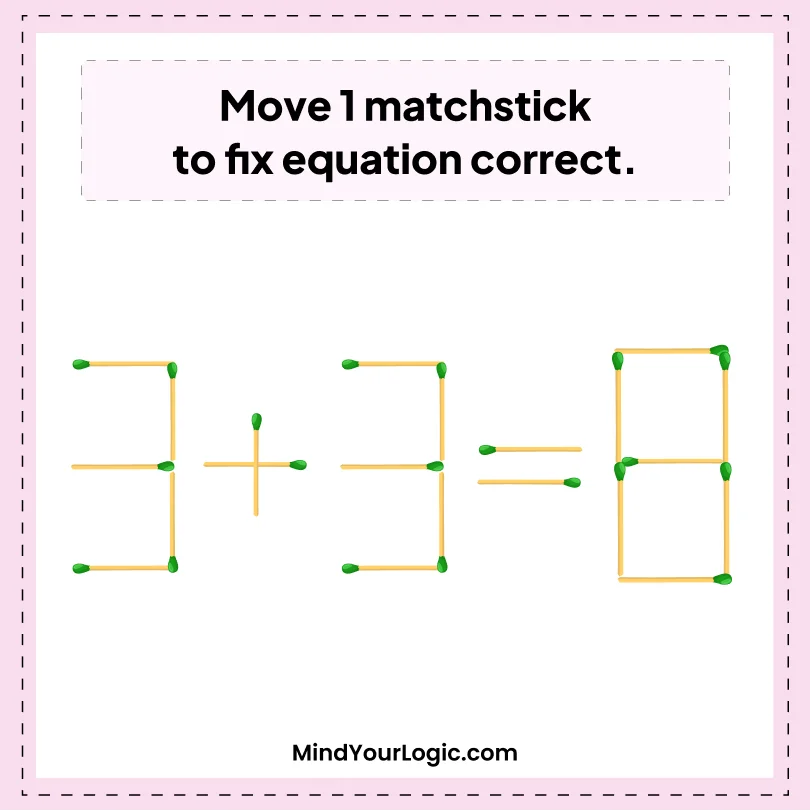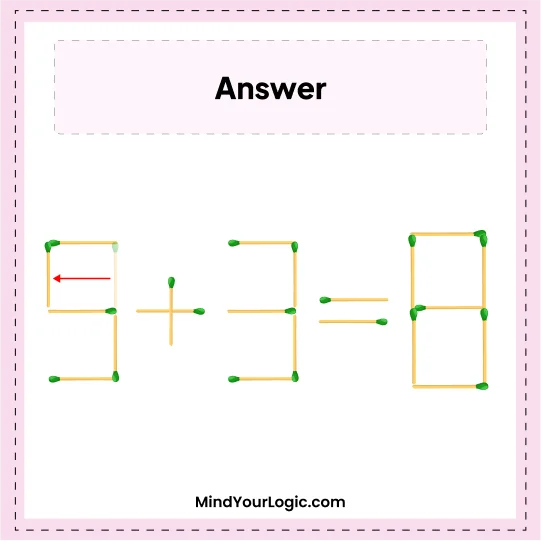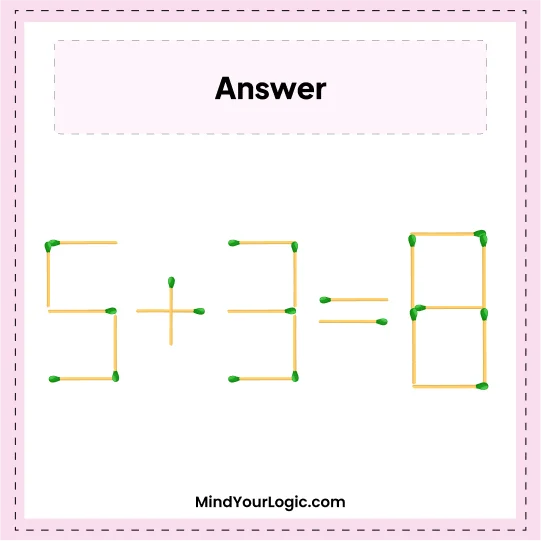# Move 1 and correct 3+3=8 - Matchstick Puzzle

###### 37.Matchstick Puzzles
`Move the  one matchstick from the following matchstick equation and try to make the below equation correct.`•

Explanation :

` Move the stick of 1st 3 and make it 5. So, we the get correct equation 5 + 3 = 8.`